# IBPS PO Quantitative Aptitude Quiz- 26

## IBPS PO Pre Quantitative Aptitude Quiz- 26

Quantitative aptitude measures a candidate’s numerical proficiency and problem-solving abilities. It is the most important section of almost all competitive exams. Candidates are often stymied by the complexity of Quantitative Aptitude Questions but if they practice more and more questions it will become quite easy. So, here we are providing you with the IBPS PO Pre Quantitative Aptitude Quiz to enhance your preparation for your upcoming examination. Questions given in this IBPS PO Pre Quantitative Aptitude Quiz are based on the most recent and the latest exam pattern. A detailed explanation for each question will be given in this IBPS PO Pre Quantitative Aptitude Quiz. This IBPS PO Pre Quantitative Aptitude Quiz is entirely free of charge. This IBPS PO Pre Quantitative Aptitude Quiz will assist aspirants in achieving a good score in their upcoming examinations.

Directions (1-5): The following graph shows the number of students participated in annual talent show of Kurukshetra university. Study the graph carefully to answer the given questions.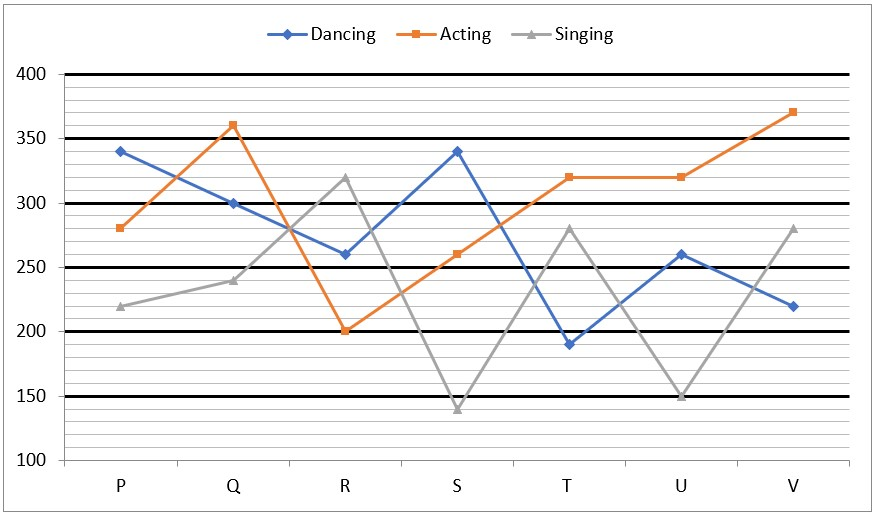1. Find the ratio of the total number of students participated in dancing from college P, T & V together to the total students participated in singing from college P, Q & R together.

(a) 28 : 27

(b) 25 : 26

(c) 25 : 29

(d) 27 : 29

(e) 25 : 31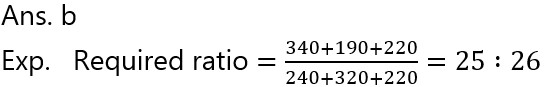1. From which of these colleges the total number of students participated is 2nd minimum?

(a) College P

(b) College Q

(c) College R

(d) College S

(e) College T1. If 40% of the total students of all colleges who participated in acting, are doing solo acting and other are in group acting. 200/3% of the students participated in group acting are a part of comedy dramas. Then find the total number of students who are acting in comedy dramas.

(a) 844

(b) 854

(c) 850

(d) 864

(e) 920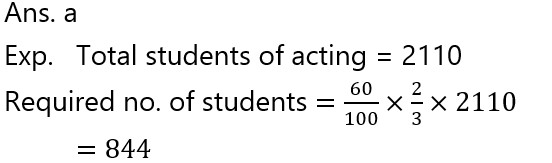1. From which college the difference between the no. of students participating in dancing and that in singing is maximum?

(a) P

(b) S

(c) T

(d) R

(e) Q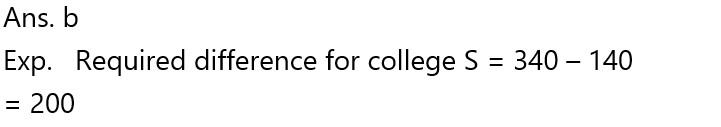1. The total number of students participated from college S is what percent more/less than the no. of that from college R?

(a) 5.23%

(b) 5.13%

(c) 5.03%

(d) 4.93%

(e) 4.73%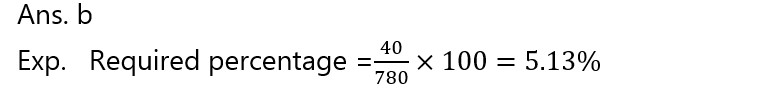Directions (6-10): In each questions two equations numbered I and II are given. You have to solve both the equations and mark the answer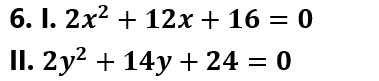(a) if x > y

(b) if x ≥ y

(c) if x< y

(d) if x ≤ y

(e) if x = y or no relation can be established between x and y.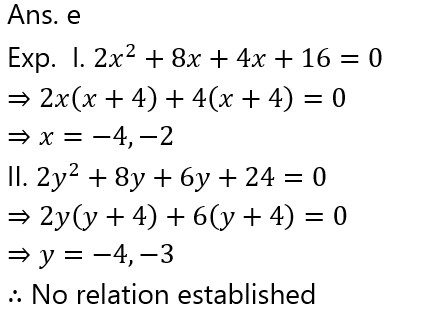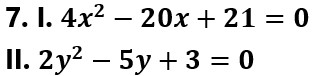(a) if x > y

(b) if x ≥ y

(c) if x< y

(d) if x ≤ y

(e) if x = y or no relation can be established between x and y.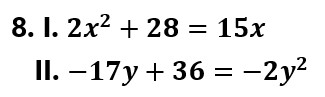(a) if x > y

(b) if x ≥ y

(c) if x< y

(d) if x ≤ y

(e) if x = y or no relation can be established between x and y.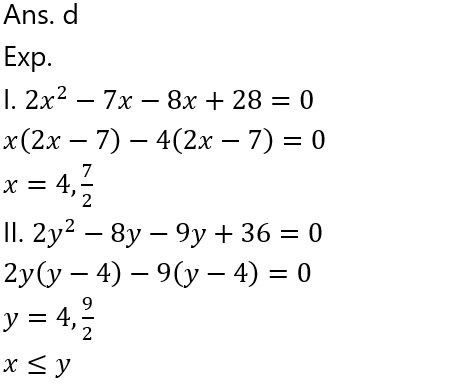(a) if x > y

(b) if x ≥ y

(c) if x< y

(d) if x ≤ y

(e) if x = y or no relation can be established between x and y.(a) if x > y

(b) if x ≥ y

(c) if x< y

(d) if x ≤ y

(e) if x = y or no relation can be established between x and y.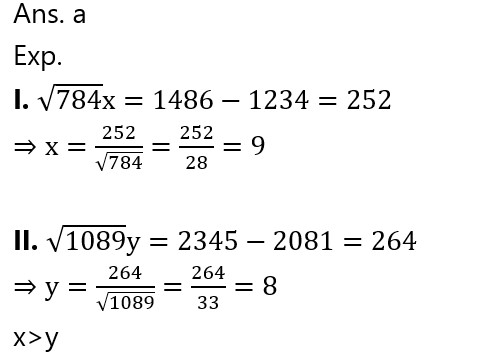###Click to Buy Bank MahaCombo Package

Recommended PDF’s for:

#### Most important PDF’s for Bank, SSC, Railway and Other Government Exam : Download PDF Now

AATMA-NIRBHAR Series- Static GK/Awareness Practice Ebook PDF Get PDF here
The Banking Awareness 500 MCQs E-book| Bilingual (Hindi + English) Get PDF here
AATMA-NIRBHAR Series- Banking Awareness Practice Ebook PDF Get PDF here
Computer Awareness Capsule 2.O Get PDF here
AATMA-NIRBHAR Series Quantitative Aptitude Topic-Wise PDF Get PDF here
AATMA-NIRBHAR Series Reasoning Topic-Wise PDF Get PDF Here
Memory Based Puzzle E-book | 2016-19 Exams Covered Get PDF here
Caselet Data Interpretation 200 Questions Get PDF here
Puzzle & Seating Arrangement E-Book for BANK PO MAINS (Vol-1) Get PDF here
ARITHMETIC DATA INTERPRETATION 2.O E-book Get PDF here

3